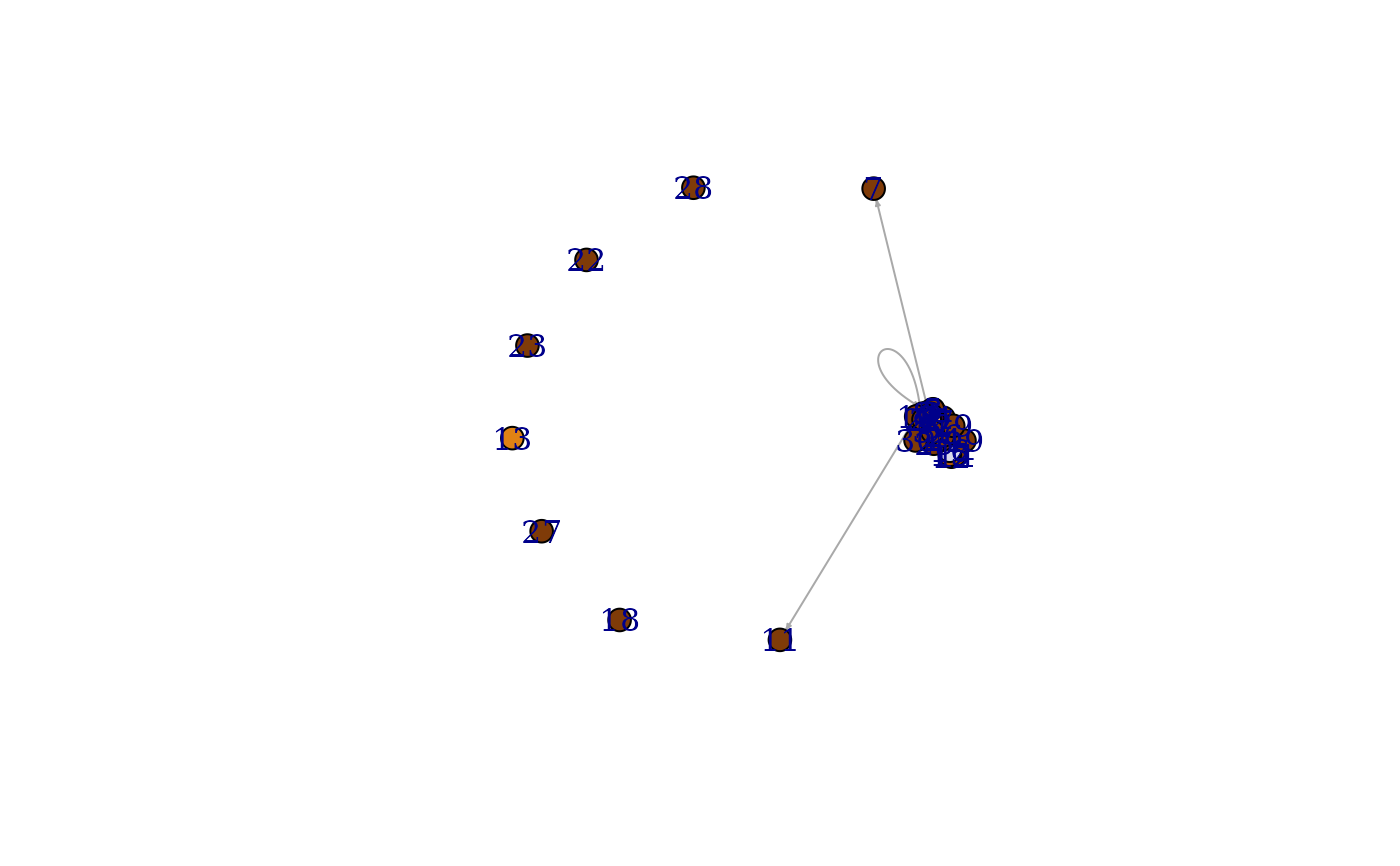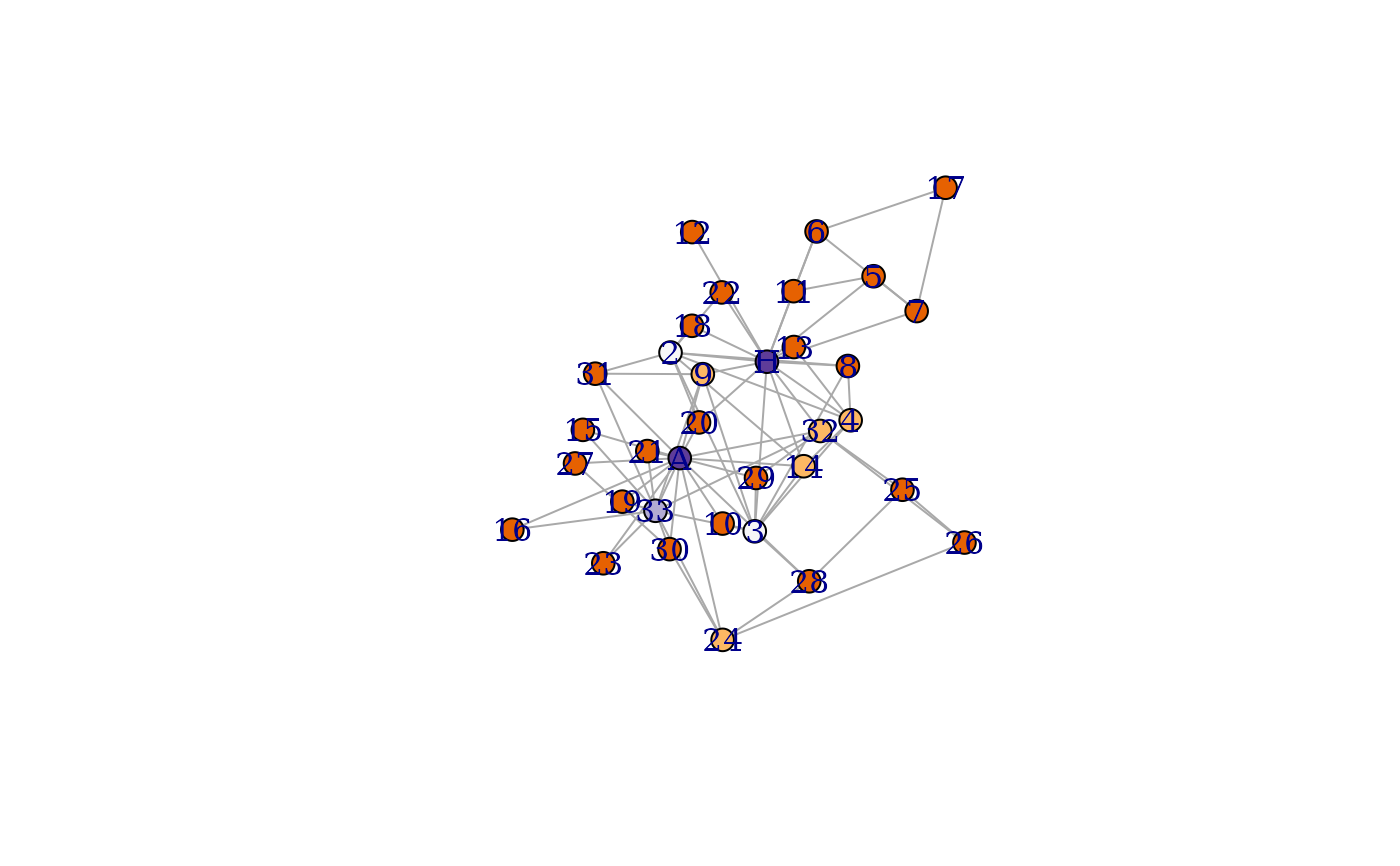This is the ‘PuOr’ palette from https://colorbrewer2.org/. It has at most eleven colors.

## Usage

diverging_pal(n)

## Arguments

n

The number of colors in the palette. The maximum is eleven currently.

## Value

A character vector of RGB color codes.

## Details

This is similar to sequential_pal(), but it also puts emphasis on the mid-range values, plus the the two extreme ends. Use this palette, if you have such a quantity to mark with vertex colors.

Other palettes: categorical_pal(), r_pal(), sequential_pal()

## Examples

library(igraphdata)
data(foodwebs)
fw <- foodwebs[] %>%
induced_subgraph(V(.)[ECO == 1]) %>%
set_vertex_attr("label", value = seq_len(gorder(.))) %>%
set_vertex_attr("size", value = 10) %>%
set_edge_attr("arrow.size", value = 0.3)
#> This graph was created by an old(er) igraph version.
#>   Call upgrade_graph() on it to use with the current igraph version
#>   For now we convert it on the fly...

V(fw)$color <- scales::dscale(V(fw)$Biomass %>% cut(10), diverging_pal)
plot(fw)data(karate)
karate <- karate %>%